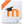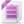Topic outline

•Mathematics Essentials Q3

Mathematics Essentials will improve student understanding of basic mathematics concepts and develop essential skills needed to be successful in the high school mathematics curriculum. The standards taught include arithmetic, algebraic reasoning, basic geometry concepts, and fundamental statistics. Math Essentials is ideally taught as a Tier 2 or Tier 3 intervention course, in conjunction with Algebra 1.•Mathematics Essentials Q3 Moodle Course File

•Mathematics Essentials Q3 Moodle Common Cartridge 1.1 Course File
•Topic 1

•WATCH: Using the Commutative and Associative Properties Page
•Topic 2

•LESSON: Solving Addition and Subtraction Equations Book

•INTERACTIVE LAB: Solving Equations using Muliplication & Division URL

•Topic 3

•LESSON: More Solving 2-Step Equations Book

•LESSON: Review Solving Equations Book

•ACTIVITY: Equation Buster Game URL
•Topic 4

•Topic 5

••Topic 6

••ACTIVITY: Math Continuum! Entertainment and Understanding Graphs Page

•ACTIVITY: Train Race (Mean, Median, and Range) URL

•ACTIVITY: Math Continuum! Entertainment and Understanding Graphs Page

•Topic 7

•Topic 8

•Topic 9

•